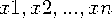Functions and CALL Routines

# LPNORM Function

Returns the Lp norm of the second argument and subsequent non-missing arguments.
 Category: Descriptive Statistics

## Syntax

 LPNORM(p, value-1 <,value-2 ...>)

### Arguments

p

specifies a numeric constant, variable, or expression that is greater than or equal to 1, which is used as the power for computing the Lp norm.

value

specifies a numeric constant, variable, or expression.

If all arguments have missing values, then the result is a missing value. Otherwise, the result is the Lp norm of the non-missing values of the second and subsequent arguments.

In the following example, p is the value of the first argument, andare the values of the other non-missing arguments.### Example 1: Calculating the Lp Norm

The following example returns the Lp norm of the second and subsequent non-missing arguments.

```data _null_;
x1 = lpnorm(1, ., 3, 0, .q, -4);
x2 = lpnorm(2, ., 3, 0, .q, -4);
x3 = lpnorm(3, ., 3, 0, .q, -4);
x999 = lpnorm(999, ., 3, 0, .q, -4);
put x1= / x2= / x3= / x999=;
run;```

SAS writes the following output to the log:

```x1=7
x2=5
x3=4.4979414453
x999=4```

### Example 2: Calculating the Lp Norm When You Use a Variable List

The following example uses a variable list and returns the Lp norm.

```data _null_;
x1 = 1;
x2 = 3;
x3 = 4;
x4 = 3;
x5 = 1;
x = lpnorm(of x1-x5);
put x=;
run;```

SAS writes the following output to the log:

`x=11`

Functions:

 SUMABS Function (L1 norm) EUCLID Function (L2 norm) MAX Function (Linfinity norm)Previous Page | Next Page | Top of Page